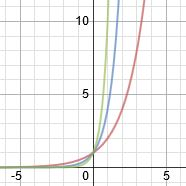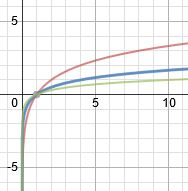# Behavior of Exponential and Logarithmic Functions

An error occurred trying to load this video.

Try refreshing the page, or contact customer support.

Coming up next: Trigonometry: Sine and Cosine

### You're on a roll. Keep up the good work!

Replay
Your next lesson will play in 10 seconds
• 0:03 Exponential Functions
• 1:45 Exponential End Behavior
• 2:20 Logarithmic Functions
• 3:48 Logarithmic End Behavior
• 4:30 Lesson Summary
Save Save

Want to watch this again later?

Timeline
Autoplay
Autoplay
Speed Speed Audio mode

#### Recommended Lessons and Courses for You

Lesson Transcript
Instructor: Yuanxin (Amy) Yang Alcocer

Amy has a master's degree in secondary education and has taught math at a public charter high school.

After watching this video lesson, you will be able to recognize exponential and logarithmic functions by looking at the end behavior of the graphs. You will also learn how the graphs change.

## Exponential Functions

What happens when you put a mama bunny together with a daddy bunny? They start to multiply, literally. Rabbits are known for how fast they make babies, and then how quickly those babies make even more babies. In math, we call this exponential growth because we can describe their growth with an exponential function.

What are these functions? They are the functions where our variable is in the exponent. That's easy to remember if you look at the first word and tell yourself that this first word is telling you where the variable is. So, we will have functions such as y = 2^x, y = 4^x, and y = 10^x. What do these look like when we graph them? Let's see:The red line is the y = 2^x graph, the blue line is the y = 4^x graph, and the green line is the y = 10^x graph. Notice how the green line actually increases faster than the blue and red lines. Why do you think this is the case? This is because the base of our exponential function is bigger. We have a 10 instead of a 2 or a 4.

If these functions showed the population growth of two different groups of rabbits, we would say that the group represented by y = 10^x, the green line, has a faster growth rate. Since these functions are representing population growth, the base of our exponential function then represents the growth factor, or how fast our population grows.

## Exponential End Behavior

What we are doing here is actually analyzing the end behavior, how our graph behaves for really large and really small values, of our graph. For exponential functions, we see that the end behavior tends to infinity really fast. The larger the growth factor, which is the base of the exponential function, the quicker we get to infinity. We also see that for very small values of our input, our variable, the graph is close to 0. For population growth, we don't worry about these values.

## Logarithmic Functions

Now, let's look at logarithmic functions and how they are different from exponential functions. They are actually related to each other. Logarithmic functions are the functions where the variable is the argument of the log function. We will see functions such as y = log base 2 (x), y = log base 4 (x), and y = log (x). Remember that for logarithmic functions, if we don't see a base number, then it is automatically 10.

I said earlier that these functions are related to our exponential functions. They are in that logarithmic functions are inverses of exponential functions. Graphed, the logarithmic version will be the mirror image of our exponential function across the line y = x. Do you want to see? Okay, here goes:To unlock this lesson you must be a Study.com Member.

### Register to view this lesson

Are you a student or a teacher?

#### See for yourself why 30 million people use Study.com

##### Become a Study.com member and start learning now.
Back
What teachers are saying about Study.com

### Earning College Credit

Did you know… We have over 200 college courses that prepare you to earn credit by exam that is accepted by over 1,500 colleges and universities. You can test out of the first two years of college and save thousands off your degree. Anyone can earn credit-by-exam regardless of age or education level.